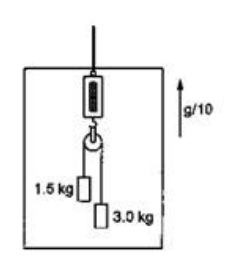Deepak Scored 45->99%ile with Bounce Back Crack Course. You can do it too!

# Solve the following :

Question:

A person is standing on a weighing machine placed on the floor of an elevator. The elevator starts going up with some acceleration, moves with uniform velocity for a while and finally decelerates to stop. The maximum and the minimum weights recorded are $72 \mathrm{~kg}$ and $60 \mathrm{~kg}$. Assuming that the magnitudes of the acceleration and the deceleration are the same, find (a) the true weight of the person and $(b)$ the magnitude of the acceleration. Take $\mathrm{g}=9.9 \mathrm{~m} / \mathrm{s}^{2}$.Solution:

$\mathrm{W}=\mathrm{W}_{\max }\left(\right.$ when $\mathrm{F}_{\mathrm{p}}$ is along $\left.\mathrm{mg}\right)$

$W=W_{\min }$ (when $F_{p}$ is opposite to $\mathrm{mg}$ )

$W_{\max }=m\left(a_{0}+g\right)=72$

$W_{\min }=m\left(-a_{0}+g\right)=60$

$\mathrm{mg}=\frac{132}{2}=$$=66 \mathrm{~kg}$
$\mathrm{a}_{0}=\frac{66-60}{6.6}=0.9 \mathrm{~m} / \mathrm{s}^{2}$